# Texas Go Math Grade 2 Lesson 12.5 Answer Key Describe Division

Refer to our Texas Go Math Grade 2 Answer Key Pdf to score good marks in the exams. Test yourself by practicing the problems from Texas Go Math Grade 2 Lesson 12.5 Answer Key Describe Division.

## Texas Go Math Grade 2 Lesson 12.5 Answer Key Describe Division

Explore

Write the answer for each step in the problem.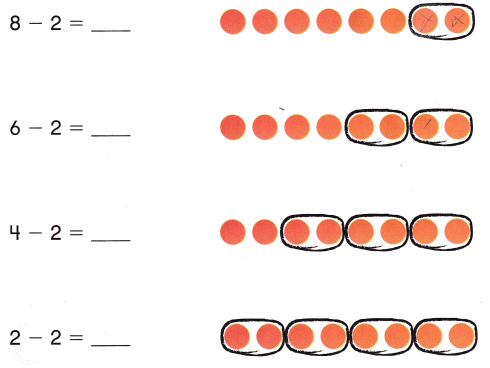FOR THE TEACHER • Read the following situation to children, Corey is making groups of two counters. He has 8 counters. How many counters are left after he makes each group? Have children complete the number sentences.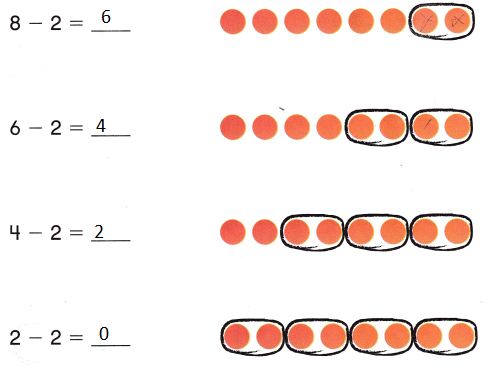Explanation:
Yes, the children completed the number sentences.
0 counters are left
after making the number sentences.

Math Talk
Mathematical Processes

How is what you did like division? Explain.
8 is divided in to 4 counters
with 2 counters each
To find the number sentence

Model and Draw

Use the pictures. Describe how the group was divided.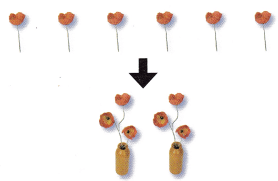There are __________ flowers.
Each vase can fit _________ flowers.
So, use __________ vases to fit all the flowers.There are __________ counters.
They are divided into __________ equal groups.
Each group has __________ counters.There are 6 flowers.
Each vase can fit 3 flowers.
So, use 2 vases to fit all the flowers.There are 10 counters.
They are divided into 5 equal groups.
Each group has 2 counters.

Share and Show

Use the pictures. Describe how the things are divided.

Question 1.Bella has __________ pictures on pages.
Each __________ can fit
So, she uses __________.
Bella has 3 pictures on pages.
Each 4 can fit
So, she uses 12.
Explanation:
3 x 4 = 12
12 ÷ 4 = 3

Question 2.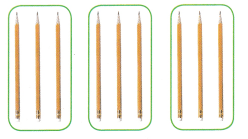There are __________ pencils.
They are divided into __________ equal groups.
So, each group has __________.
There are 9 pencils.
They are divided into 3 equal groups.
So, each group has 3.
Explanation:
In total there are 9 pencils
each group has 3 pencils
shared among 3 groups

Problem Solving

Use the pictures. Describe how the things are divided.

Question 3.Jack has __________ cookies on plates.
Each ___________.
So, he uses _____________.
Jack has 8 cookies on plates.
Each 4 .
So, he uses 2.
Explanation:
In total there are 8 cookies
they were shared among 2 plates
each plate has 4 cookies each

Question 4.There are __________ shells. They are divided into _________________.
So, ____________________
There are 4 shells. They are divided into 2.
So, 2
Explanation:
In total there are 4 shells
They are shared among 2 groups
4 ÷ 2 = 2
Each group has 2 shells

Solve. Write or draw to explain.

Question 5.
H.O.T. Multi-Step Chase has 20 pebbles and Mia has 7 pebbles. They divide all the pebbles into groups of 3. How many equal groups are there?____________ equal groups
9 equal groups
Explanation:
Chase has 20 pebbles and Mia has 7 pebbles.
20 + 7 = 27
They divide all the pebbles into groups of 3.
27 ÷ 3 = 9
9 equal groups are there

Question 6.
H.O.T. Lily buys 6 bags of dog treats. Each bag has the same number of treats. She buys 24 treats in all. How many treats are in each bag?
___________ treats
4 treats
Explanation:
Lily buys 6 bags of dog treats.
Each bag has the same number of treats.
She buys 24 treats in all.
24 ÷ 6 = 4
4 treats are in each bag

Question 7.
Reasoning There are 14- puppets in a puppet show. One person controls 2 puppets. How many people are controlling the puppets?
(A) 6
(B) 8
(C) 7
Explanation:
There are 14- puppets in a puppet show.
One person controls 2 puppets.
14 ÷ 2 = 7
7 people are controlling the puppets

Question 8.
Use the pictures to describe how things are divided.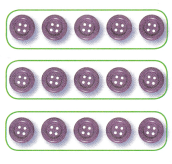There are ___________ buttons.
They are divided into ______________.
So, _______________.
There are 15 buttons.
They are divided into 3.
So, 5.
Explanation:
In total there are 15 buttons
they are shared among 3 groups
and 5 in each group.

Question 9.
TEXAS Test Prep Cole has 21 toy cars. He divides them into 3 equal groups. How many toy cars are in each group?
(A) 18
(B) 24
(C) 7
Explanation:
Cole has 21 toy cars.
He divides them into 3 equal groups.
21 ÷ 3 = 7
7 toy cars are in each group

TAKE HOME ACTIVITY • Ask your child to show how he or she solved an exercise in the lesson.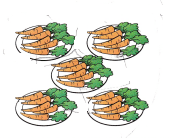Betty has ____________ carrots on plates.
Each _____________.
So, she uses ______________.
Betty has 25 carrots on plates.
Each 5 carrots
So, she uses 5 plates.
Explanation:
25 carrots are shared among 5 plates
25 ÷5 = 5
so, 5 plates

### Texas Go Math Grade 2 Lesson 12.5 Homework and Practice Answer Key

Use the pictures. Describe how the things are divided.

Question 1.Betty has ____________ carrots on plates.
Each _____________.
So, she uses ______________.
Betty has 25 carrots on plates.
Each 5 carrots
So, she uses 5 plates.
Explanation:
25 carrots are shared among 5 plates
25 ÷5 = 5
so, 5 plates

Question 2.There are 6 oranges. They are divided into ______________ .
So, _____________________.
There are 6 oranges. They are divided into 3.
So, 2. in each box
Explanation:
6 ÷ 3 = 2
6 oranges shared in 3 boxes
Each box has 2 oranges each

Problem Solving

Solve. Write or draw to explain.

Question 3.
Jordan buys 4 boxes of postcards. He buys 28 postcards in all. How many postcards are in each box?
__________ postcards
7 postcards
Explanation:
Jordan buys 4 boxes of postcards.
He buys 28 postcards in all.
28 ÷ 4 = 7
7 postcards are in each box

Question 4.
Multi-Step Carlo has 12 daisies and 6 roses. She divides the flowers into groups of 3. How many flowers are in each group?
_____________ groups
6 flowers
Explanation:
Carlo has 12 daisies and 6 roses.
12 + 6 = 18
She divides the flowers into groups of 3.
18 ÷ 3 = 6
6 flowers are in each group

Lesson Check

Question 5.
Greg has 27 toy trucks. He divides them into 3 equal groups. How many toy trucks are in each group?
(A) 9
(B) 7
(C) 8
Explanation:
Greg has 27 toy trucks.
He divides them into 3 equal groups.
27 ÷ 3 = 9
9 toy trucks are in each group

Question 6.
Sandy has a bag of 35 peanuts. She gave 5 friends an equal number of peanuts. How many peanuts did each friend get?
(A) 8
(B) 6
(C) 7
Explanation:
Sandy has a bag of 35 peanuts.
She gave 5 friends an equal number of peanuts.
35 ÷ 5 = 7
7 peanuts that each friend get

Question 7.
The flower shop has 32 flowers in vases. Each vase has 8 flowers in it. How many vases are there?
(A) 4
(B) 7
(C) 6
Explanation:
The flower shop has 32 flowers in vases.
Each vase has 8 flowers in it.
32 ÷ 8 = 4
4 vases are there

Question 8.
The lunch room has 6 tables and 36 chairs. There are an equal number of chairs at each table. How many chairs are at each table?
(A) 7
(B) 6
(C) 5